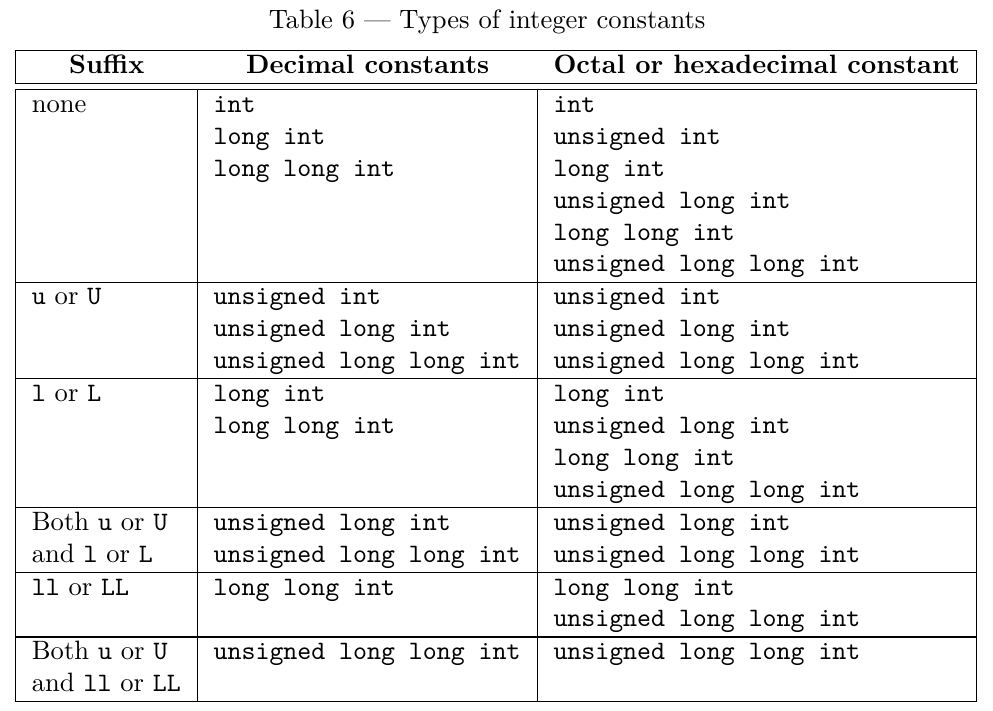# What’s the difference between 0xffffffff and 0xffffffffu?

In C++, what is the difference between 0xffffffff and 0xffffffffu?

This one’s pretty easy to answer with this information from the C++ standard:

The type of an integer literal is the first of the corresponding list in Table 6 in which its value can be represented.0xffffffff is a hexadecimal constant, it’s too big to be represented in a (signed) int, so — by the terms of the standard — the type of 0xffffffff is unsigned int.

Furthermore, each of these hexadecimal literals will have a different type:

```0x7fffffff   // int
0xffffffff   // unsigned int
0x1ffffffff  // long int (or long long int)
0x1ffffffffu // unsigned long int (or unsigned long long int)
```

But to answer the original question, there is no difference between 0xffffffff and 0xffffffffu apart from this: